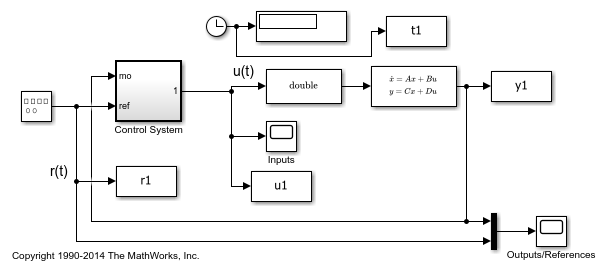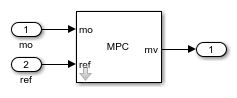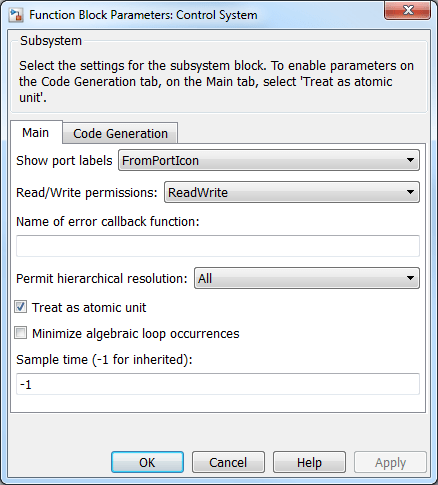# Simulation and Structured Text Generation Using Simulink PLC Coder

This example shows how to simulate and generate Structured Text for an MPC Controller block using Simulink® PLC Coder™ software. The generated code uses single-precision.

### Required Products

```if ~mpcchecktoolboxinstalled('simulink') disp('Simulink is required to run this example.') return end if ~mpcchecktoolboxinstalled('plccoder') disp('Simulink PLC Coder is required to run this example.'); return end ```

### Setup Environment

You must have write-permission to generate the relevant files and the executable. Therefore, before starting simulation and code generation, change the current directory to a temporary directory.

```cwd = pwd; tmpdir = tempname; mkdir(tmpdir); cd(tmpdir); ```

### Define Plant Model and MPC Controller

Define a SISO plant.

```plant = ss(tf([3 1],[1 0.6 1])); ```

Define the MPC controller for the plant.

```Ts = 0.1; %Sample time p = 10; %Prediction horizon m = 2; %Control horizon Weights = struct('MV',0,'MVRate',0.01,'OV',1); % Weights MV = struct('Min',-Inf,'Max',Inf,'RateMin',-100,'RateMax',100); % Input constraints OV = struct('Min',-2,'Max',2); % Output constraints mpcobj = mpc(plant,Ts,p,m,Weights,MV,OV); ```

### Simulate and Generate Structured Text

```mdl = 'mpc_plcdemo'; open_system(mdl) ```To generate structured text for the MPC Controller block, complete the following two steps:

• Configure the MPC block to use single-precision data. Set the Output data type property of the MPC Controller block to `single`.

```open_system([mdl '/Control System/MPC Controller']) ```• Put the MPC block inside a subsystem block and treat the subsystem block as an atomic unit. Select the Treat as atomic unit property of the subsystem block.```close_system([mdl '/Control System/MPC Controller']) open_system([mdl '/Outputs//References']) open_system([mdl '/Inputs']) sim(mdl) ```
```-->Converting model to discrete time. -->Assuming output disturbance added to measured output channel #1 is integrated white noise. -->The "Model.Noise" property of the "mpc" object is empty. Assuming white noise on each measured output channel. ```To generate code with the PLC Coder, use the `plcgeneratecode` command.

```disp('Generating PLC structure text... Please wait until it finishes.') plcgeneratecode([mdl '/Control System']); ```
```Generating PLC structure text... Please wait until it finishes. ### Generating PLC code for 'mpc_plcdemo/Control System'. ### Using <a href="matlab:configset.showParameterGroup('mpc_plcdemo', { 'PLC Code Generation' } )">model settings</a> from 'mpc_plcdemo' for PLC code generation parameters. ### Begin code generation for IDE <a href="matlab:configset.showParameterGroup('mpc_plcdemo', { 'PLC Code Generation' } )">codesys23</a>. ### Emit PLC code to file. ### Creating PLC code generation report <a href="matlab:web('/tmp/Bdoc21b_1757077_260767/tp83fb630a_6576_4b43_9b77_bca43ba3bf4d/plcsrc/html/mpc_plcdemo/mpc_plcdemo_codegen_rpt.html')">mpc_plcdemo_codegen_rpt.html</a>. ### PLC code generation successful for 'mpc_plcdemo/Control System'. ### Generated files: <a href="matlab: edit('plcsrc/mpc_plcdemo.exp')">plcsrc/mpc_plcdemo.exp</a> ```

The Message Viewer dialog box shows that PLC code generation was successful.```bdclose(mdl) cd(cwd) ```